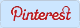# Math Trivia Worksheets

 Home Number Sense Pre-Algebra Algebra Geometry Statistics Math Test Membership Login## Math Trivia: Questions and Answers

 Math Trivia is information or math facts about a particular concept. In this page we have presented you some of the math facts. Answers are given at the end. Kids, parents and teachers can make use of these free printable math trivia or math facts worksheets. Math facts worksheets include information about numbers such as counting numbers, whole numbers, integers and complex numbers, decimals, fractions, percents, polynomials, geometry concepts such as points, straight lines, circles, triangles, polygons and many more.

 Natural numbers start at 1 and goes to infinity. The difference between two consecutive integers is 1. In set notation, N = {1, 2, 3, 4, ...}
 Math Facts on Integers: Integers include natural numbers, negative of a natural numbers and zero. Zero takes the center position. Z = {..., -3, -2, -1, 0, 1, 2, 3, ...}
 Math Trivia on Whole Numbers 1: Whole numbers are set of natural numbers with an additional element zero. Whole numbers are represented by W. W = {0, 1, 2, 3, ...}
 Math Trivia on Whole Numbers 2: Learn about least, greatest whole numbers in different digits, difference between them, product and more facts.